# NUMERICAL SIMULATION OF LIQUID SLOSHING IN LNG TANK USING GPU-ACCELERATED MPS METHOD1)

Chen Xiang, Wan Decheng2)Collaborative Innovation Center for Advanced Ship and Deep-Sea Exploration, State Key Laboratory of Ocean Engineering, School of Naval Architecture, Ocean and Civil Engineering, Shanghai Jiao Tong University, Shanghai 200240, China

Abstract

Liquid sloshing is a common phenomenon induced in partially filled tanks under external excitations, which may destroy the tank structure and vessel stability. Moving particle semi-implicit (MPS) method is a typical meshfree method which can effectively simulate violent liquid sloshing problem. However, the low computational efficiency of MPS is the bottleneck of its application in large-scale three-dimensional problems. In the past years, GPU parallel acceleration technique has been widely used in the field of scientific computing. In this work, GPU parallel acceleration technique is introduced into MPS method and an in-house solver MPSGPU-SJTU is developed by using CUDA language. Then this solver is used to simulate 3-D liquid sloshing in liquefied natural gas (LNG) tank. The convergent validation of particle spacing is carried out to verify the accuracy of present solver. The maximum particle number of simulation model is over two million particles. MPSGPU-SJTU solver can accurately predict the impact pressures by comparing with other results. In addition, the violent flow phenomena such as large deformation and nonlinear fragmentation of free surface can be observed in these simulations. The comparison of computation time between GPU and CPU solvers demonstrates GPU parallel acceleration technique can significantly reduce the computation time and improve the computational efficiency of MPS. The phenomena of liquid sloshing in LNG tank and rectangular tank are compared. The results show that LNG tank can reduce the sloshing amplitude and impact pressure in high filling level. However, the sloshing is more violent and the free surface presents three-dimensional feature in LNG tank with middle and low filling level. Finally, the investigation of the effect of different fluids such as water and LNG on sloshing phenomena is also conducted in this paper. It shows that the flow fields of both liquids are almost similar and the impact pressure is proportional to the liquid density.

Keywords： meshfree particle method ; MPS method ; parallel acceleration technique ; liquid sloshing

0

Chen Xiang, Wan Decheng. NUMERICAL SIMULATION OF LIQUID SLOSHING IN LNG TANK USING GPU-ACCELERATED MPS METHOD1)[J]. Chinese Journal of Theoretical and Applied Mechanics, 2019, 51(3): 714-729 https://doi.org/10.6052/0459-1879-18-410

## 引 言

Faltinsen最早推导出了在水平激励作用下的二维液舱晃荡的解析解.Nakayama和Washizu[2-3]分别用有 限元方法和边界元方法分析了二维液舱晃荡问题.Kim等采用标记网格法对二维和三维薄膜型液舱的晃荡问题进行了模拟,并分析了各参数对计算结果产生的影响.Zhu等应用改进的等值面函数法对大幅度液舱晃荡的波高和砰击压力进行了准确的预报.Xue和Lin采用流体体积法模拟了三维矩形液舱的晃荡问题,并对环形隔板的减晃效果进行了分析.虽然在液舱晃荡问题中,网格类方法已经取得了许多成果,但在处理强非线性变化的自由液面时仍有一定的困难,如波浪破碎、自由面翻卷等.无网格粒子类方法基于拉格朗日方法描述,粒子之间不存在固定的拓扑关系,能够很方便地模拟物体和自由液面的非线性变形[7-9].在液舱晃荡数值模拟方面,Shao等[10-11]对光滑粒子流体动力学法进行了改进,提高了数值精度,对横荡和横摇激励作用下的液舱晃荡进行了研究,并讨论了各种隔板类型的减晃效果.Zhang和Liu提出了解耦有限粒子法,通过数值测试验证了该方法的准确性,并将其用于与二维液舱晃荡模拟.移动粒子半隐式法(moving particle semi-implicit,MPS)作为一种典型的粒子类方法也已广泛应用于液舱晃荡问题中,成为分析研究该问题不可或缺的技术手段.

Rueda等应用MPS模拟了在波浪环境中,带流体液舱的运动响应,并通过傅里叶变换得到的幅值响应算子与实验比对吻合. 潘徐杰等基于改进的MPS,比较了不同自由面条件、核函数对液舱晃荡数值模拟的影响.Lee等用MPS方法模拟了在外流作用下带流体液舱的运动响应,并与无流体液舱的运动响应进行了对比,结果表明液舱内的流体能有效地减小液舱的运动响应.Tsukamoto等在二维液舱中间布置了一个浮体,浮体与舱壁之间用弹簧连接,并用MPS方法研究了在不同充液率和激励频率下,流体对浮体的作用力.Zhang等应用改进的MPS方法对二维液舱晃荡问题进行了数值模拟,并将计算结果与实验和网格类方法结果进行了对比,壁面砰击压力和自由面波形吻合较好.杨亚强应用改进MPS方法对液舱晃荡进行了系统研究,包括激励频率和充液率对晃荡的影响、不同自由度激励下的晃荡特征、二维三维矩形或薄膜形液舱的晃荡,以及水平或垂直隔板对砰击压力和自由面破碎的抑制作用等.Zhang等[19-20]将MPS与有限元方法结合,研究了弹性液舱内的晃荡现象以及弹性隔板对晃荡的抑制效果.Wen等[21-22]开发了多相流MPS方法,并将该方法应用到双层和三层流体的晃荡问题中,计算波高和砰击压力与实验结果吻合较好.

## 1 MPS数值方法

MPS方法是一种典型的基于拉格朗日描述流场的无网格粒子类方法,它最先由Koshizuka和Oka在1996年提出,并用于模拟二维溃坝问题.该方法不需要离散N-S方程的对流项,可以有效地避免对流项离散而引起的数值发散问题,同时计算过程不需要使用网格,省去了网格生成与重构的繁杂过程,并且该方法易于追踪捕捉大变形或强非线性破碎的自由面.鉴于以上优点,越来越多的研究人员对MPS进行了深入研究,改进其数值模型提高计算精度,并将其应用于剧烈流动问题.在此,将简要介绍本文所采用的MPS方法的数值模型.

### 1.1 控制方程

$\nabla \cdot {\pmb V} = \dfrac{1}{\rho }\dfrac{{\rm D}\rho }{{\rm D}t} = 0 (1)$

$\dfrac{{\rm D}{\pmb V}}{{\rm D}t} = - \dfrac{1}{\rho }\nabla P + \nu \nabla ^2{\pmb V} + {\pmb g} (2)$

### 1.2 核函数

$W\left( r \right) = \left\{ \!\!\begin{array}{ll} \dfrac{r_{\rm e} }{r} -1\,,& 0 \leqslant r < r_{\rm e} 0\,,& r_{\rm e} \leqslant r \end{array} \right. (3)$

$W\left( r \right) = \left\{ \begin{array}{ll} \dfrac{r_{\rm e} }{0.85r + 0.15r_{\rm e} } -1\,, & 0 \leqslant r < r_{\rm e} 0\,, & r_{\rm e} \leqslant r \end{array} \right. (4)$

### 1.3 粒子作用模型

MPS方法中,控制方程中的微分算子都是基于粒子模型来进行离散的. 本文将介绍MPS方法中几个重要的粒子模型：梯度模型 (式(5))、散度模型 (式(6))和拉普拉斯模型 (式(7))

$\langle \nabla \phi \rangle_i = \dfrac{D}{n^0}\sum_{j \ne i} \dfrac{\phi _j + \phi _i }{ | {\pmb r}_j - {\pmb r}_i |^2} ( {\pmb r}_j - {\pmb r}_i ) \cdot W(| {\pmb r}_j - {\pmb r}_i | ) (5)$

$\langle \nabla \cdot {\pmb V} \rangle_i = \dfrac{D}{n^0}\sum_{j \ne i} \dfrac{({\pmb V}_j - {\pmb V}_i ) \cdot ({\pmb r}_j - {\pmb r}_i )}{| {\pmb r}_j - {\pmb r}_i |^2} \cdot W(| {\pmb r}_j - {\pmb r}_i | ) (6)$

$\langle \nabla ^2\phi \rangle_i = \dfrac{2D}{n^0\lambda }\sum_{j \ne i} (\phi _j - \phi _i ) \cdot W(| {\pmb r}_j - {\pmb r}_i | ) (7)$

$\lambda = \dfrac{\sum_{j \ne i} W(|{\pmb r}_j - {\pmb r}_i |) \cdot |{\pmb r}_j - {\pmb r}_i |^2} {\sum_{j \ne i} W(| {\pmb r}_j - {\pmb r}_i | )} (8)$

### 1.4 不可压缩模型

$\langle \nabla ^2P^{k + 1} \rangle_i = - \dfrac{\rho }{\Delta t^2}\dfrac{ \langle n^\ast \rangle _i - n^0}{n^0} (9)$

$\langle n \rangle _i = \sum_{j \ne i} W\left( {\left| {\pmb r}_j - {\pmb r}_i \right|} \right) (10)$

$\langle \nabla ^2P^{k + 1} \rangle _i = (1 - \gamma )\dfrac{\rho }{\Delta t}\nabla \cdot {\pmb V}_i^\ast - \gamma \dfrac{\rho }{\Delta t^2}\dfrac{ \langle n^\ast \rangle _i - n^0}{n^0} (11)$

### 1.5 自由面粒子判断

$$\langle n \rangle _i < \beta \cdot n^0 (12)$$

${\pmb F}_i = \dfrac{D}{n^0}\sum_{j\ne i} \dfrac{1}{| {\pmb r}_i - {\pmb r}_j |} ({\pmb r}_i - {\pmb r}_j )W(r_{ij} ) (13)$

$\left| {\pmb F} \right| > 0.9\left| {\pmb F} \right|^0 (14)$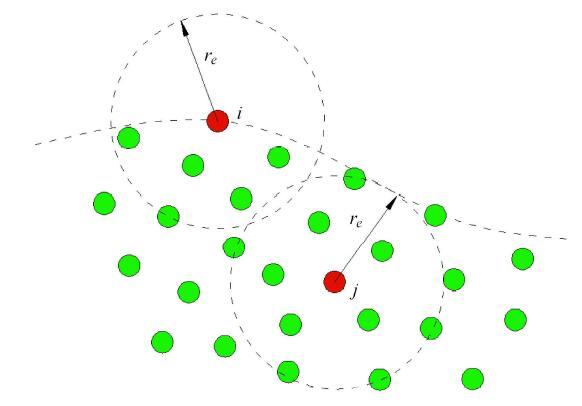Fig. 1   Free surface detection

### 1.6 边界条件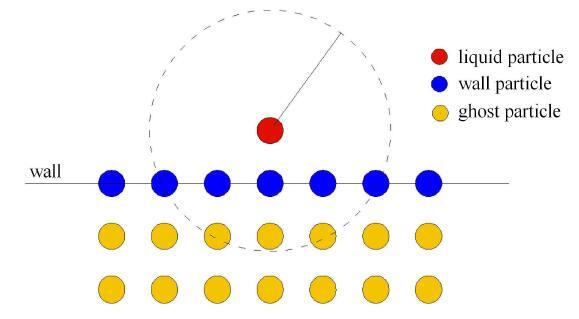Fig. 2   Schematic of boundary particles

### 1.7 计算流程

MPS方法采用半隐式方法求解流体的压力和速度. 每一个时间步迭代主要包含预测和修正两个部分. 第一步是根据重力和黏性力计算出流体的临时速度,如式(15)和式(16).第二步是由式(11)计算得到粒子的压力,根据压力场计算修正流体的速度和更新粒子的位置,如式(17)和 式(18)

$\Delta {\pmb V}_i^\ast = \Delta t\left( {\nu \nabla ^2{\pmb V}_i^n + {\pmb g}} \right) (15)$

${\pmb V}_i^\ast = {\pmb V}_i^n + \Delta {\pmb V}_i^\ast (16)$

${\pmb V}_i^{n + 1} = {\pmb V}_i^\ast - \Delta t\dfrac{1}{\rho }\nabla P^{n + 1} (17)$

${\pmb r}_i^{n + 1} = {\pmb r}_i^n + \Delta t{\pmb V}_i^{n + 1} (18)$

## 2 GPU并行加速技术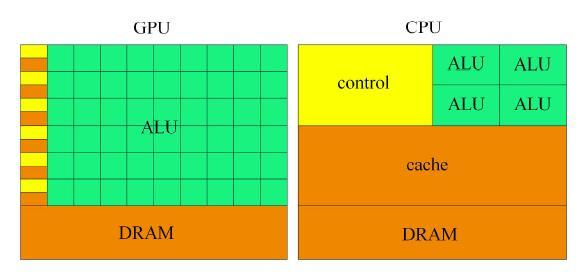Fig. 3   The hardware frameworks of GPU and CPU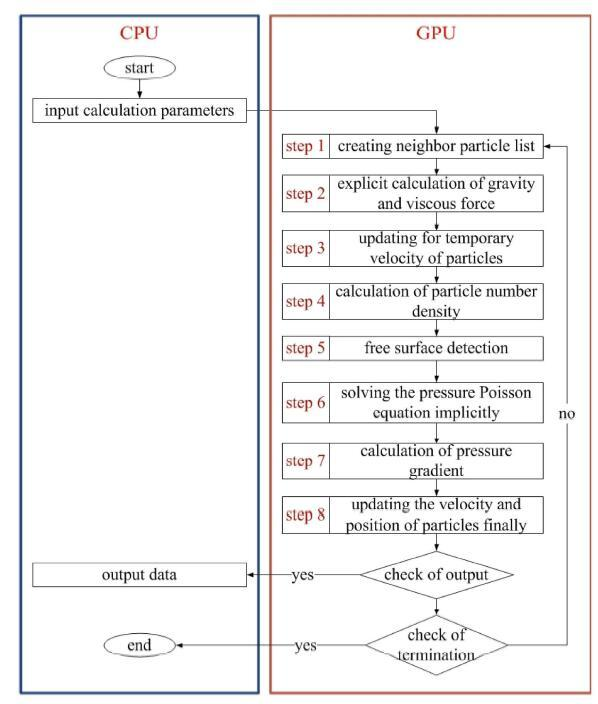Fig. 4   The flow chart of MPS on GPU

(1)划分背景网格,搜寻邻居粒子,建立邻居粒子表.

(2)采用显式方法计算黏性力和重力.

(3)更新粒子的临时速度.

(4)计算粒子数密度.

(5)判断自由面粒子.

(6)构建压力泊松方程,采用隐式方法计算流场压力.

(7)插值得到第二类边界粒子压力,计算压力梯度.

(8)更新粒子速度和位置.

## 3 数值模拟

Table 1   The computing environment of HPC cluster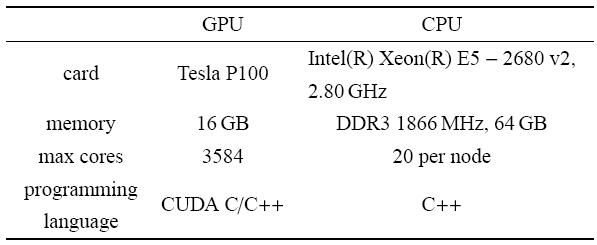$$\theta = \theta _0 \cdot \sin (\omega \cdot t) (19)$$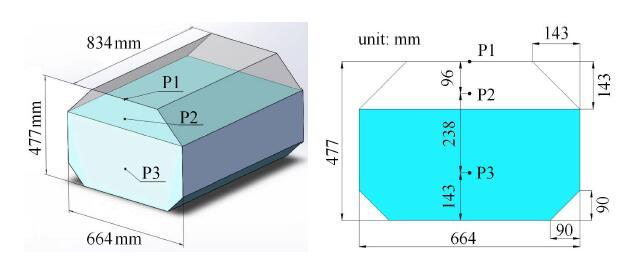Fig. 5   Schematic of LNG tank

### 3.1 粒子间距收敛性验证

Table 2   Computational parameters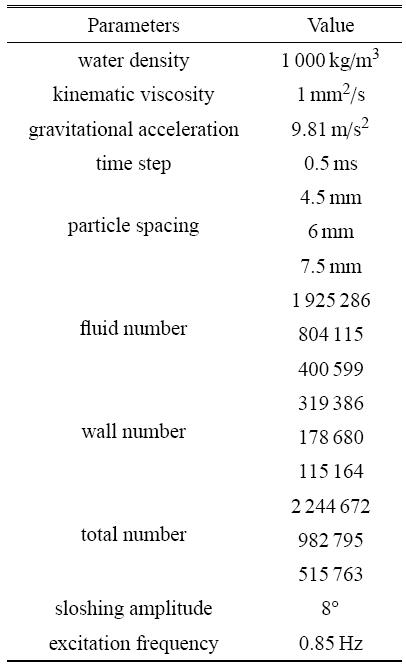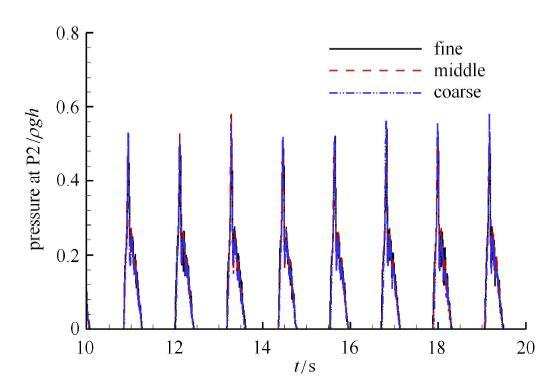Fig. 6   The impact pressure at P2 of different particle resolutions

Table 3   The impact pressure at P2 of different particle resolutions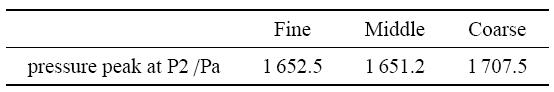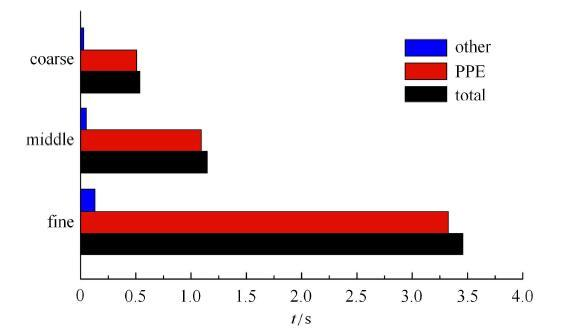Fig. 7   The computation times of different particle resolutions

Table 4   The computation times of different particle resolutions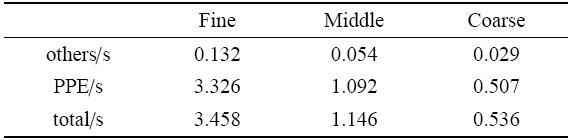### 3.2 数值结果验证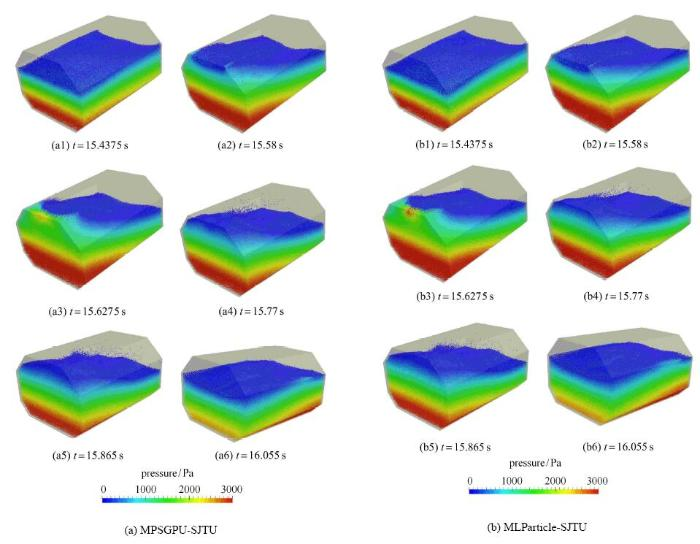Fig. 8   The flow fields of liquid sloshing by GPU and CPU simulations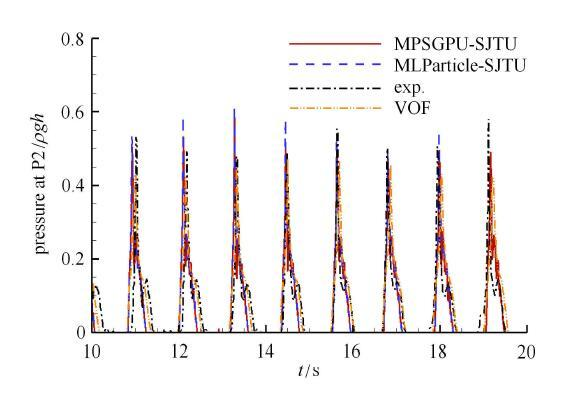Fig. 9   The impact pressure at P2 of different researches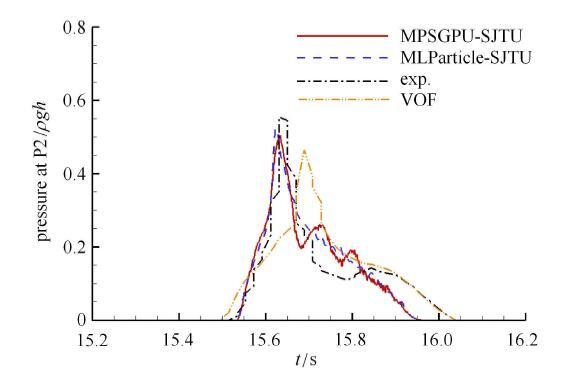Fig. 10   The enlarged signals of impact pressure at P2

$$r_{\rm s} = \dfrac{t_{\rm CPU} }{t_{\rm GPU} } (20)$$

Table 5   The computation times of GPU and CPU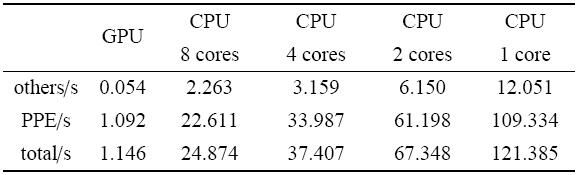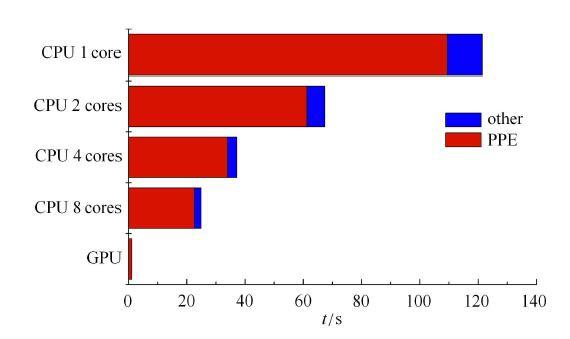Fig. 11   The computation times of GPU and CPU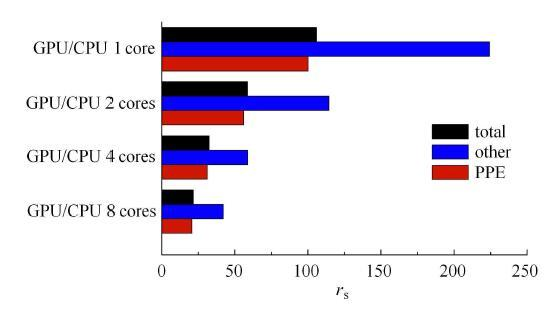Fig. 12   The speedup ratio of GPU

### 3.3 不同液舱构型晃荡对比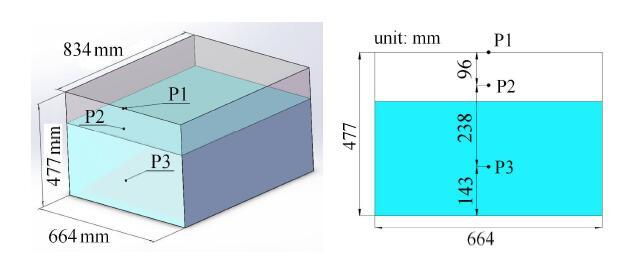Fig. 13   Schematic of rectangular tank

3.3.1 70%充液率对比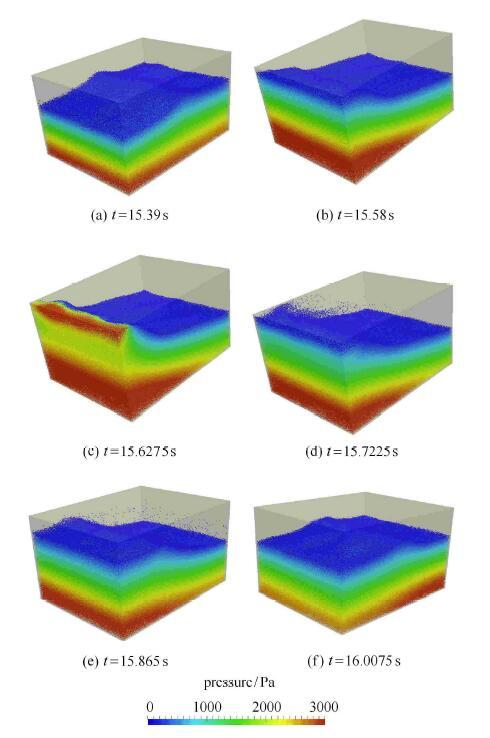Fig. 14   The flow fields of liquid sloshing in rectangular tank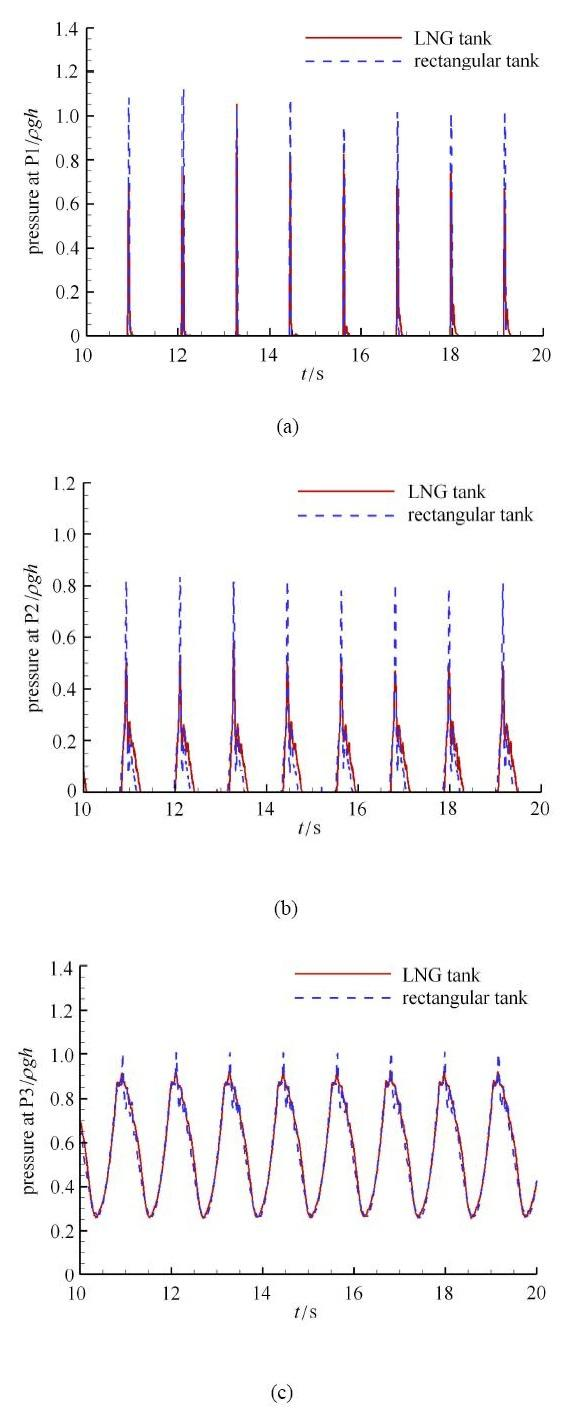Fig. 15   The impact pressure in different tanks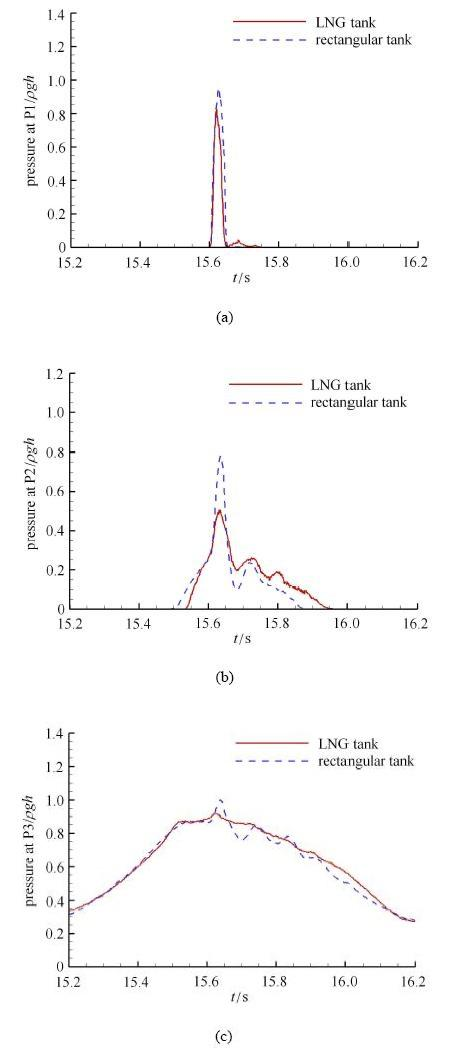Fig.16   The enlarged signals of impact pressure

3.3.2 50%充液率对比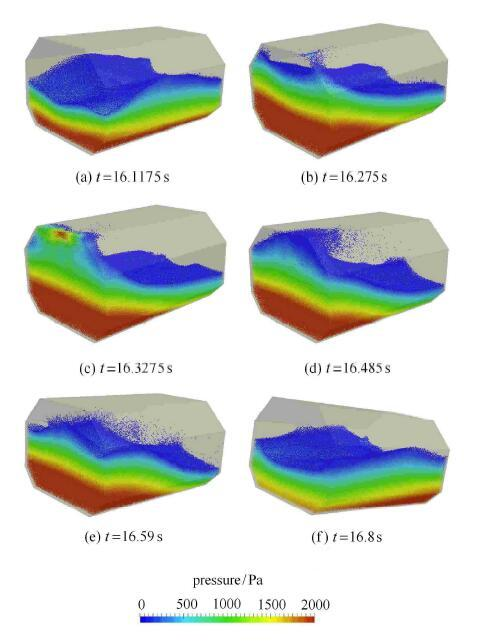Fig. 17   The flow fields of liquid sloshing in LNG tank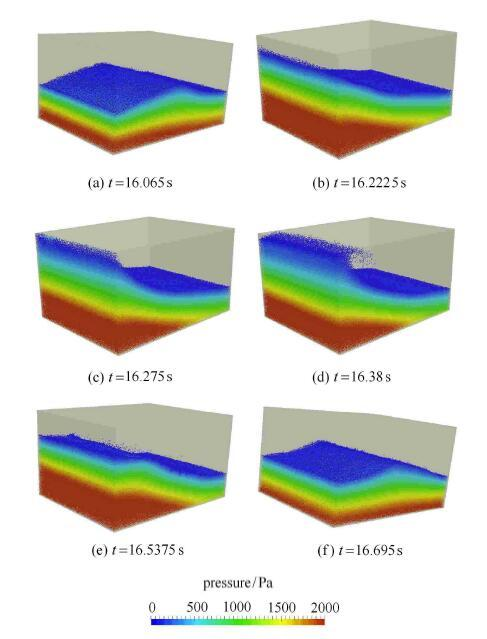Fig. 18   The flow fields of liquid sloshing in rectangular tank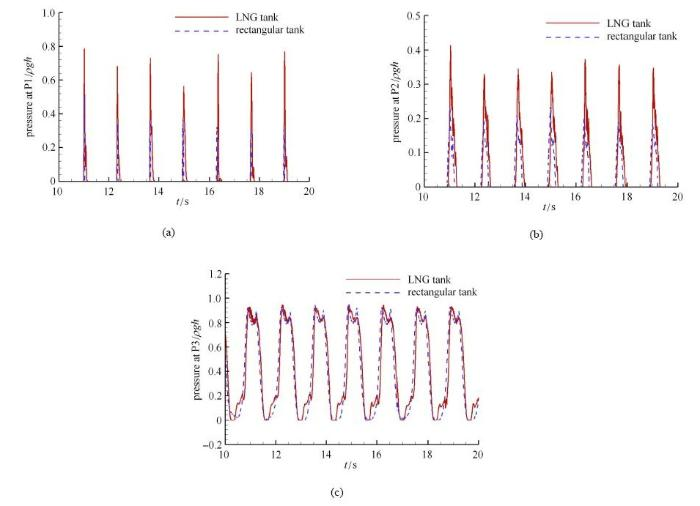Fig. 19   The impact pressure in different tanks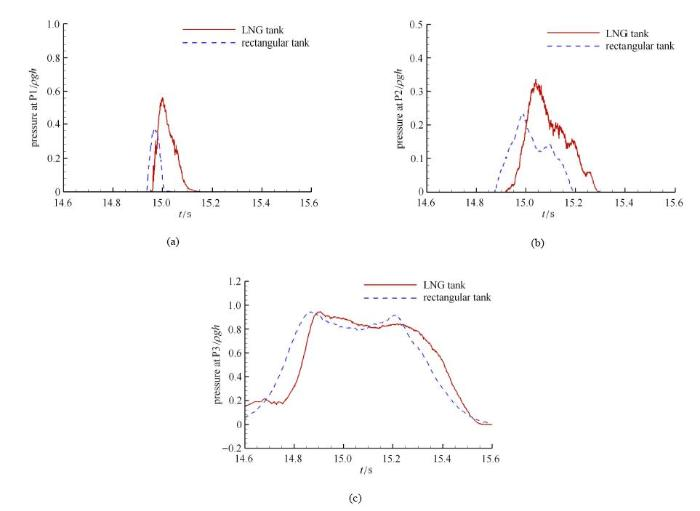Fig. 20   The enlarged signals of impact pressure

3.3.3 30%充液率对比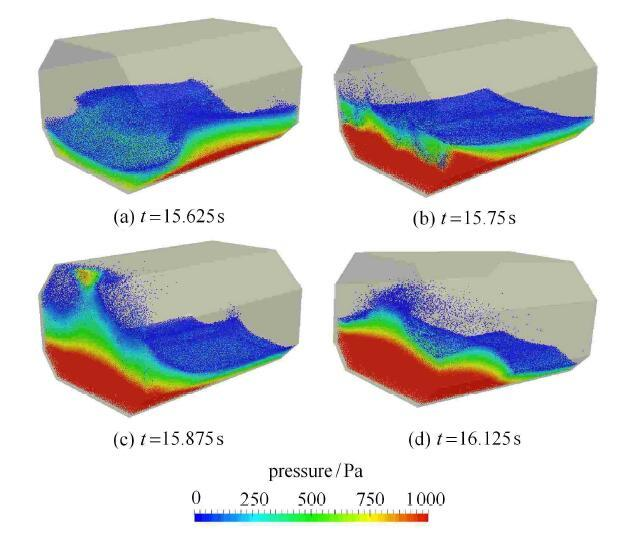Fig. 21   The flow fields of liquid sloshing in LNG tank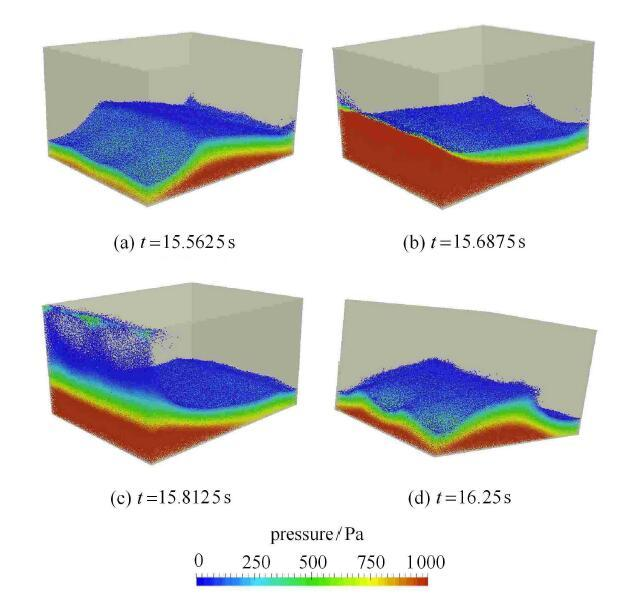Fig. 22   The flow fields of liquid sloshing in rectangular tank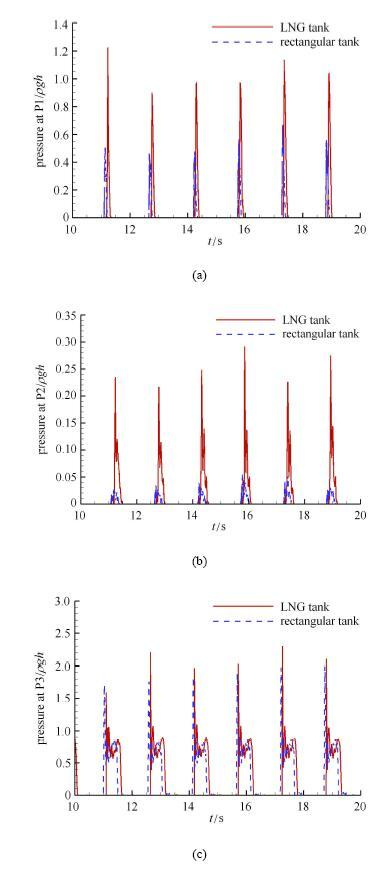Fig. 23   The impact pressure in different tanks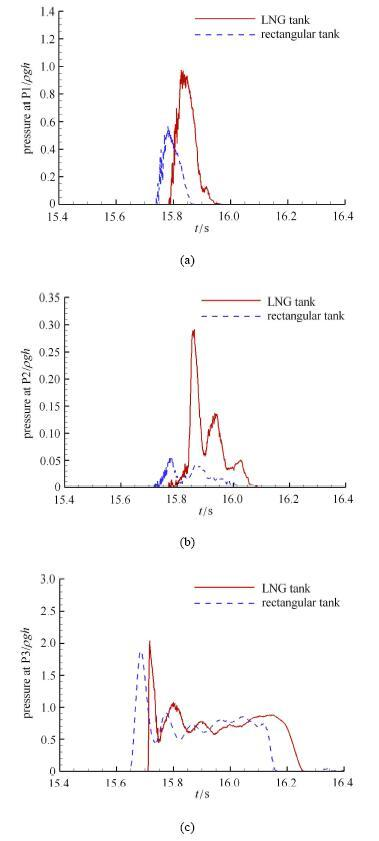Fig. 24   The enlarged signals of impact pressure

### 3.4 不同液体密度晃荡对比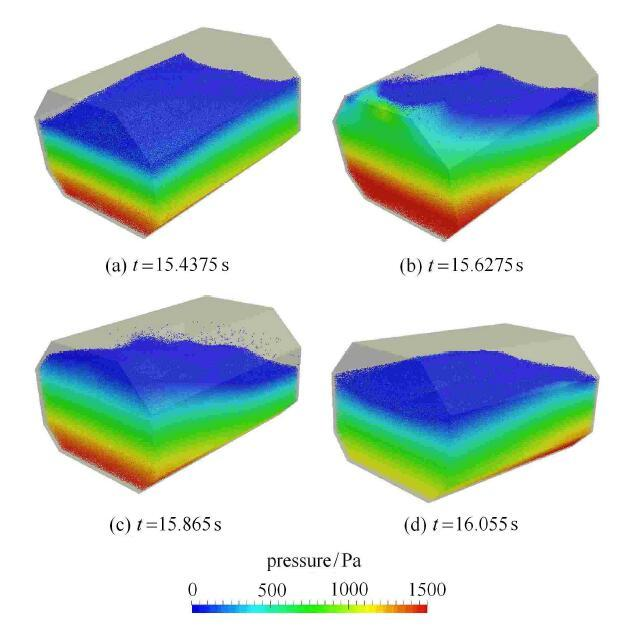Fig. 25   The flow fields of liquid sloshing of liquefied natural gas

$$\dfrac{pressure_{water} }{pressure_{LNG} } = \dfrac{density_{water} }{density_{LNG} } (21)$$

Table 6   The impact pressure of different fluid densities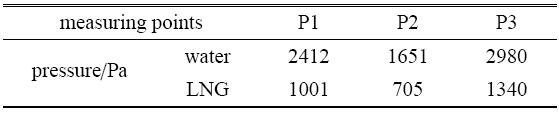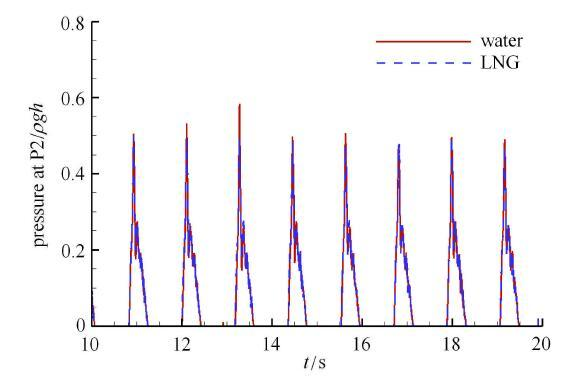Fig. 26   The impact pressure at P2 of different fluid densities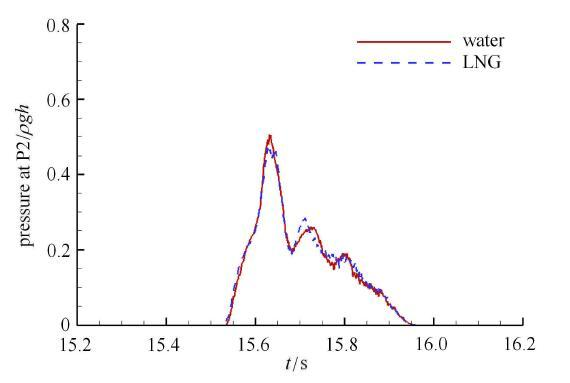Fig. 27   The enlarged signals of impact pressure at P2

## 4 结论

The authors have declared that no competing interests exist.

## 参考文献 原文顺序 文献年度倒序 文中引用次数倒序 被引期刊影响因子〈〉